# ChainLadder: Claims reserving with R

#### 2022-01-09

Abstract

The ChainLadder package provides various statistical methods which are typically used for the estimation of outstanding claims reserves in general insurance, including those to estimate the claims development results as required under Solvency II.

To cite package 'ChainLadder' in publications use:

Markus Gesmann, Daniel Murphy, Yanwei (Wayne) Zhang, Alessandro
Carrato, Mario Wuthrich, Fabio Concina and Eric Dal Moro (2022).
ChainLadder: Statistical Methods and Models for Claims Reserving in
https://mages.github.io/ChainLadder/.

## Introduction

### Claims reserving in insurance

The insurance industry, unlike other industries, does not sell products as such but promises. An insurance policy is a promise by the insurer to the policyholder to pay for future claims for an upfront received premium.

As a result insurers don’t know the upfront cost for their service, but rely on historical data analysis and judgement to predict a sustainable price for their offering. In General Insurance (or Non-Life Insurance, e.g. motor, property and casualty insurance) most policies run for a period of 12 months. However, the claims payment process can take years or even decades. Therefore often not even the delivery date of their product is known to insurers.

In particular losses arising from casualty insurance can take a long time to settle and even when the claims are acknowledged it may take time to establish the extent of the claims settlement cost. Claims can take years to materialize. A complex and costly example are the claims from asbestos liabilities, particularly those in connection with mesothelioma and lung damage arising from prolonged exposure to asbestos. A research report by a working party of the Institute and Faculty of Actuaries estimated that the un-discounted cost of UK mesothelioma-related claims to the UK Insurance Market for the period 2009 to 2050 could be around £10bn, see (Gravelsons et al. 2009). The cost for asbestos related claims in the US for the worldwide insurance industry was estimate to be around 120bn in 2002, see (Michaels 2002). Thus, it should come as no surprise that the biggest item on the liability side of an insurer’s balance sheet is often the provision or reserves for future claims payments. Those reserves can be broken down in case reserves (or outstanding claims), which are losses already reported to the insurance company and losses that are incurred but not reported (IBNR) yet. Historically, reserving was based on deterministic calculations with pen and paper, combined with expert judgement. Since the 1980’s, with the arrival of personal computer, spreadsheet software became very popular for reserving. Spreadsheets not only reduced the calculation time, but allowed actuaries to test different scenarios and the sensitivity of their forecasts. As the computer became more powerful, ideas of more sophisticated models started to evolve. Changes in regulatory requirements, e.g. Solvency II in Europe, have fostered further research and promoted the use of stochastic and statistical techniques. In particular, for many countries extreme percentiles of reserve deterioration over a fixed time period have to be estimated for the purpose of capital setting. Over the years several methods and models have been developed to estimate both the level and variability of reserves for insurance claims, see (Schmidt 2017) or (England and Verrall 2002) for an overview. In practice the Mack chain-ladder and bootstrap chain-ladder models are used by many actuaries along with stress testing / scenario analysis and expert judgement to estimate ranges of reasonable outcomes, see the surveys of UK actuaries in 2002, (Lyons et al. 2002), and across the Lloyd’s market in 2012, (Orr 2012). ## The ChainLadder package ### Motivation The ChainLadder package provides various statistical methods which are typically used for the estimation of outstanding claims reserves in general insurance. The package started out of presentations given by Markus Gesmann at the Stochastic Reserving Seminar at the Institute of Actuaries in 2007 and 2008, followed by talks at Casualty Actuarial Society (CAS) meetings joined by Dan Murphy in 2008 and Wayne Zhang in 2010. Implementing reserving methods in R has several advantages. R provides: • a rich language for statistical modelling and data manipulations allowing fast prototyping • a very active user base, which publishes many extensions • many interfaces to data bases and other applications, such as MS Excel • an established framework for End User Computing, including documentation, testing and workflows with version control systems • code written in plain text files, allowing effective knowledge transfer • an effective way to collaborate over the internet • built in functions to create reproducible research reports • in combination with other tools such as LaTeX or RMarkdown easy to set up automated reporting facilities • access to academic research, which is often first implemented in R ### Brief package overview This vignette will give the reader a brief overview of the functionality of the ChainLadder package. The functions are discussed and explained in more detail in the respective help files and examples, see also (Gesmann 2014). A set of demos is shipped with the packages and the list of demos is available via: demo(package="ChainLadder") ### Installation You can install ChainLadder in the usual way from CRAN, e.g.: install.packages('ChainLadder') For more details about installing packages see (R Development Core Team 2022b). ## Using the ChainLadder package ### Working with triangles Historical insurance data is often presented in form of a triangle structure, showing the development of claims over time for each exposure (origin) period. An origin period could be the year the policy was written or earned, or the loss occurrence period. Of course the origin period doesn’t have to be yearly, e.g. quarterly or monthly origin periods are also often used. The development period of an origin period is also called age or lag. Data on the diagonals present payments in the same calendar period. Note, data of individual policies is usually aggregated to homogeneous lines of business, division levels or perils. Most reserving methods of the ChainLadder package expect triangles as input data sets with development periods along the columns and the origin period in rows. The package comes with several example triangles. The following R command will list them all: library(ChainLadder) data(package="ChainLadder") Let’s look at one example triangle more closely. The following triangle shows data from the Reinsurance Association of America (RAA): RAA  dev origin 1 2 3 4 5 6 7 8 9 10 1981 5012 8269 10907 11805 13539 16181 18009 18608 18662 18834 1982 106 4285 5396 10666 13782 15599 15496 16169 16704 NA 1983 3410 8992 13873 16141 18735 22214 22863 23466 NA NA 1984 5655 11555 15766 21266 23425 26083 27067 NA NA NA 1985 1092 9565 15836 22169 25955 26180 NA NA NA NA 1986 1513 6445 11702 12935 15852 NA NA NA NA NA 1987 557 4020 10946 12314 NA NA NA NA NA NA 1988 1351 6947 13112 NA NA NA NA NA NA NA 1989 3133 5395 NA NA NA NA NA NA NA NA 1990 2063 NA NA NA NA NA NA NA NA NA This triangle shows the known values of loss from each origin year and of annual evaluations thereafter. For example, the known values of loss originating from the 1988 exposure period are 1351, 6947, and 13112 as of year ends 1988, 1989, and 1990, respectively. The latest diagonal – i.e., the vector 18834, 16704, $$\dots$$ 2063 from the upper right to the lower left – shows the most recent evaluation available. The column headings – 1, 2,$$\dots$$, 10 – hold the ages (in years) of the observations in the column relative to the beginning of the exposure period. For example, for the 1988 origin year, the age of the 13112 value, evaluated as of 1990-12-31, is three years. The objective of a reserving exercise is to forecast the future claims development in the bottom right corner of the triangle and potential further developments beyond development age 10. Eventually all claims for a given origin period will be settled, but it is not always obvious to judge how many years or even decades it will take. We speak of long and short tail business depending on the time it takes to pay all claims. #### Plotting triangles The first thing you often want to do is to plot the data to get an overview. For a data set of class triangle the ChainLadder package provides default plotting methods to give a graphical overview of the data: plot(RAA/1000, main = "Claims development by origin year")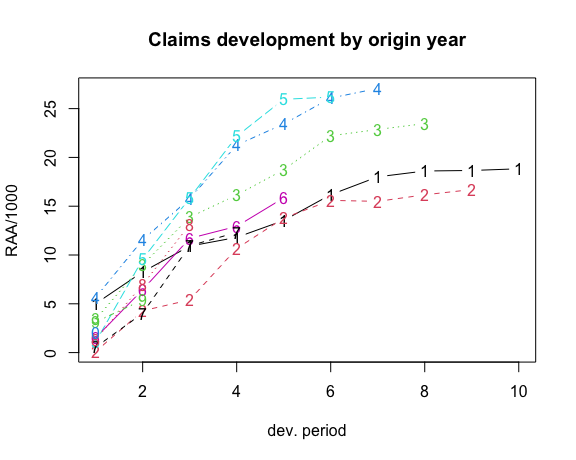Claims development chart of the RAA triangle, with one line per origin period. Setting the argument lattice=TRUE will produce individual plots for each origin period. plot(RAA/1000, lattice=TRUE, main = "Claims development by origin year")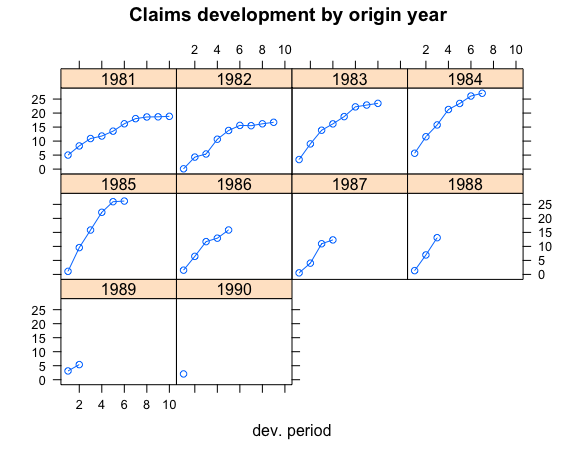Claims development chart of the RAA triangle, with individual panels for each origin period You will notice from the plots that the triangle RAA presents claims developments for the origin years 1981 to 1990 in a cumulative form. For more information on the triangle plotting functions see the help pages of plot.triangle. ### Transforming triangles between cumulative and incremental representation The ChainLadder packages comes with two helper functions, cum2incr and incr2cum to transform cumulative triangles into incremental triangles and vice versa: raa.inc <- cum2incr(RAA) ## Show first origin period and its incremental development raa.inc[1,]  1 2 3 4 5 6 7 8 9 10 5012 3257 2638 898 1734 2642 1828 599 54 172  raa.cum <- incr2cum(raa.inc) ## Show first origin period and its cumulative development raa.cum[1,]  1 2 3 4 5 6 7 8 9 10 5012 8269 10907 11805 13539 16181 18009 18608 18662 18834  #### Importing triangles from external data sources In most cases you want to analyse your own data, usually stored in data bases or spreadsheets. ##### Importing a triangle from a spreadsheet There are many ways to import the data from a spreadsheet. A quick and dirty solution is using a CSV-file. Open a new workbook and copy your triangle into cell A1, with the first column being the accident or origin period and the first row describing the development period or age. Ensure the triangle has no formatting, such a commas to separate thousands, as those cells will be saved as characters.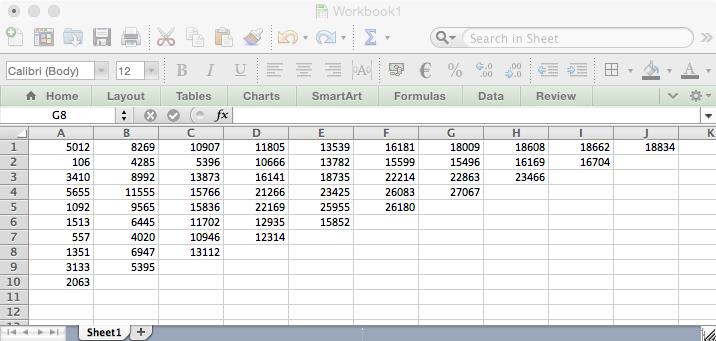Screen shot of a triangle in a spreadsheet software. Now open R and go through the following commands: myCSVfile <- "path/to/folder/with/triangle.csv" ## Use the R command: # myCSVfile <- file.choose() to select the file interactively tri <- read.csv(file=myCSVfile, header = FALSE) ## Use read.csv2 if semicolons are used as a separator likely ## to be the case if you are in continental Europe library(ChainLadder) ## Convert to triangle tri <- as.triangle(as.matrix(tri)) # Job done. Small data sets can be transferred to R backwards and forwards via the clipboard under MS Windows. Select a data set in the spreadsheet and copy it into the clipboard, then go to R and type: tri <- read.table(file="clipboard", sep="\t", na.strings="") ##### Reading data from a data base R makes it easy to access data using SQL statements, e.g. via an ODBC connection1, for more details see (R Development Core Team 2022a). The ChainLadder packages includes a demo to showcase how data can be imported from a MS Access data base, see: demo(DatabaseExamples) In this section we use data stored in a CSV-file2 to demonstrate some typical operations you will want to carry out with data stored in data bases. CSV stands for comma separated values, stored in a text file. Note many European countries use a comma as decimal point and a semicolon as field separator, see also the help file to read.csv2. In most cases your triangles will be stored in tables and not in a classical triangle shape. The ChainLadder package contains a CSV-file with sample data in a long table format. We read the data into R’s memory with the read.csv command and look at the first couple of rows and summarise it: filename <- file.path(system.file("Database", package="ChainLadder"), "TestData.csv") myData <- read.csv(filename) head(myData)  origin dev value lob 1 1977 1 153638 ABC 2 1978 1 178536 ABC 3 1979 1 210172 ABC 4 1980 1 211448 ABC 5 1981 1 219810 ABC 6 1982 1 205654 ABC summary(myData)  origin dev value lob Min. : 1 Min. : 1.00 Min. : -17657 Length:701 1st Qu.: 3 1st Qu.: 2.00 1st Qu.: 10324 Class :character Median : 6 Median : 4.00 Median : 72468 Mode :character Mean : 642 Mean : 4.61 Mean : 176632 3rd Qu.:1979 3rd Qu.: 7.00 3rd Qu.: 197716 Max. :1991 Max. :14.00 Max. :3258646  Let’s focus on one subset of the data. We select the RAA data again: raa <- subset(myData, lob %in% "RAA") head(raa)  origin dev value lob 67 1981 1 5012 RAA 68 1982 1 106 RAA 69 1983 1 3410 RAA 70 1984 1 5655 RAA 71 1985 1 1092 RAA 72 1986 1 1513 RAA To transform the long table of the RAA data into a triangle we use the function as.triangle. The arguments we have to specify are the column names of the origin and development period and further the column which contains the values: raa.tri <- as.triangle(raa, origin="origin", dev="dev", value="value") raa.tri  dev origin 1 2 3 4 5 6 7 8 9 10 1981 5012 3257 2638 898 1734 2642 1828 599 54 172 1982 106 4179 1111 5270 3116 1817 -103 673 535 NA 1983 3410 5582 4881 2268 2594 3479 649 603 NA NA 1984 5655 5900 4211 5500 2159 2658 984 NA NA NA 1985 1092 8473 6271 6333 3786 225 NA NA NA NA 1986 1513 4932 5257 1233 2917 NA NA NA NA NA 1987 557 3463 6926 1368 NA NA NA NA NA NA 1988 1351 5596 6165 NA NA NA NA NA NA NA 1989 3133 2262 NA NA NA NA NA NA NA NA 1990 2063 NA NA NA NA NA NA NA NA NA We note that the data has been stored as an incremental data set. As mentioned above, we could now use the function incr2cum to transform the triangle into a cumulative format. We can transform a triangle back into a data frame structure: raa.df <- as.data.frame(raa.tri, na.rm=TRUE) head(raa.df)  origin dev value 1981-1 1981 1 5012 1982-1 1982 1 106 1983-1 1983 1 3410 1984-1 1984 1 5655 1985-1 1985 1 1092 1986-1 1986 1 1513 This is particularly helpful when you would like to store your results back into a data base. The following figure gives you an idea of a potential data flow between R and data bases.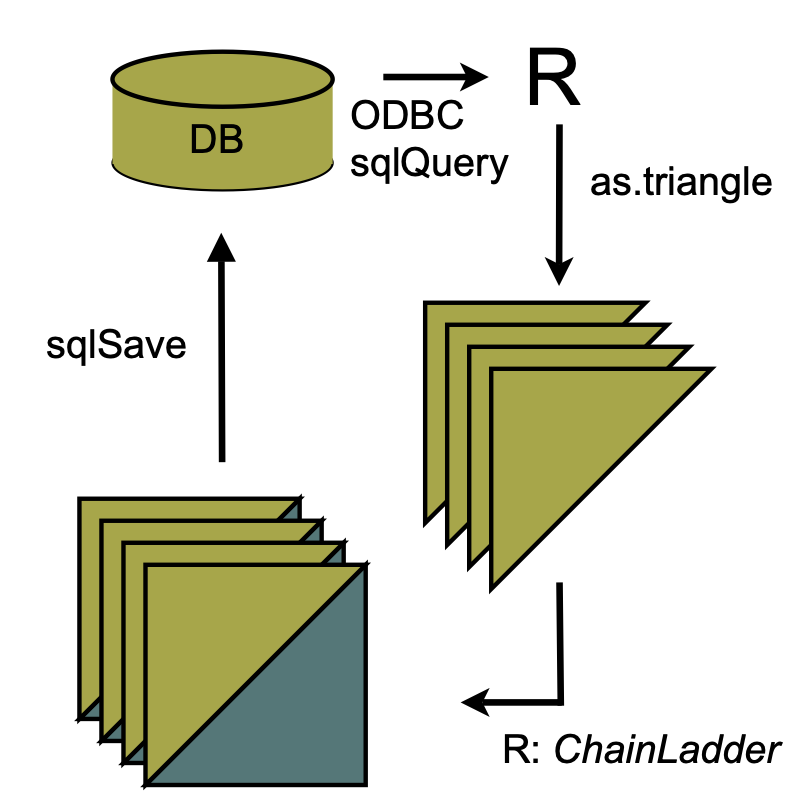Flow chart of data between R and data bases #### Creating triangles interactively For small data sets or while testing procedures, it may be useful to create triangles interactively from the command line. There are two main ways to proceed. With the first we create a matrix of data (including missing values in the lower right portion of the triangle) and then convert it into a triangle with as.triangle: as.triangle(matrix(c(100, 150, 175, 180, 200, 110, 168, 192, 205, NA, 115, 169, 202, NA, NA, 125, 185, NA, NA, NA, 150, NA, NA, NA, NA), nrow = 5, byrow = TRUE))  dev origin 1 2 3 4 5 1 100 150 175 180 200 2 110 168 192 205 NA 3 115 169 202 NA NA 4 125 185 NA NA NA 5 150 NA NA NA NA We may also create the triangle directly with triangle by providing the rows (or columns) of known data as vectors, thereby omitting the missing values: triangle(c(100, 150, 175, 180, 200), c(110, 168, 192, 205), c(115, 169, 202), c(125, 185), 150)  dev origin 1 2 3 4 5 1 100 150 175 180 200 2 110 168 192 205 NA 3 115 169 202 NA NA 4 125 185 NA NA NA 5 150 NA NA NA NA ## Chain-ladder methods The classical chain-ladder is a deterministic algorithm to forecast claims based on historical data. It assumes that the proportional developments of claims from one development period to the next are the same for all origin years. ### Basic idea Most commonly as a first step, the age-to-age link ratios are calculated as the volume weighted average development ratios of a cumulative loss development triangle from one development period to the next $$C_{ik}, i,k =1, \dots, n$$. \begin{aligned} f_{k} &= \frac{\sum_{i=1}^{n-k} C_{i,k+1}}{\sum_{i=1}^{n-k}C_{i,k}} \end{aligned} # Calculate age-to-age factors for RAA triangle n <- 10 f <- sapply(1:(n-1), function(i){ sum(RAA[c(1:(n-i)),i+1])/sum(RAA[c(1:(n-i)),i]) } ) f  2.999 1.624 1.271 1.172 1.113 1.042 1.033 1.017 1.009 Often it is not suitable to assume that the oldest origin year is fully developed. A typical approach is to extrapolate the development ratios, e.g. assuming a linear model on a log scale. dev.period <- 1:(n-1) plot(log(f-1) ~ dev.period, main="Log-linear extrapolation of age-to-age factors") tail.model <- lm(log(f-1) ~ dev.period) abline(tail.model)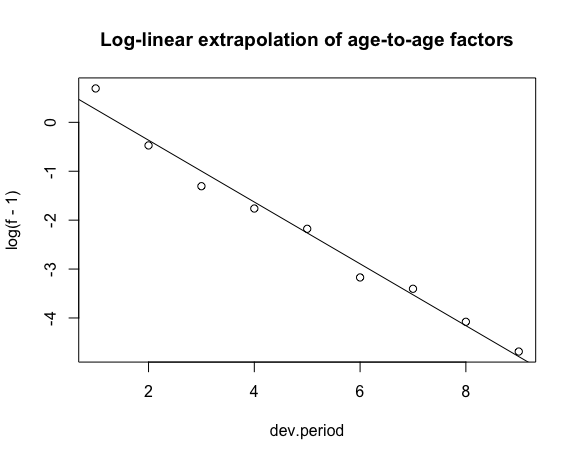co <- coef(tail.model) ## extrapolate another 100 dev. period tail <- exp(co + c(n:(n + 100)) * co) + 1 f.tail <- prod(tail) f.tail  1.009 The age-to-age factors allow us to plot the expected claims development patterns. plot(100*(rev(1/cumprod(rev(c(f, tail[tail>1.0001]))))), t="b", main="Expected claims development pattern", xlab="Dev. period", ylab="Development % of ultimate loss")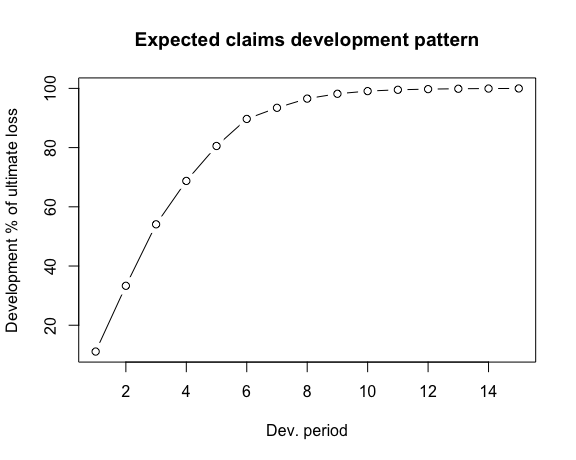The link ratios are then applied to the latest known cumulative claims amount to forecast the next development period. The squaring of the RAA triangle is calculated below, where an ultimate column is appended to the right to accommodate the expected development beyond the oldest age (10) of the triangle due to the tail factor (1.009) being greater than unity. f <- c(f, f.tail) fullRAA <- cbind(RAA, Ult = rep(0, 10)) for(k in 1:n){ fullRAA[(n-k+1):n, k+1] <- fullRAA[(n-k+1):n,k]*f[k] } round(fullRAA)  1 2 3 4 5 6 7 8 9 10 Ult 1981 5012 8269 10907 11805 13539 16181 18009 18608 18662 18834 19012 1982 106 4285 5396 10666 13782 15599 15496 16169 16704 16858 17017 1983 3410 8992 13873 16141 18735 22214 22863 23466 23863 24083 24311 1984 5655 11555 15766 21266 23425 26083 27067 27967 28441 28703 28974 1985 1092 9565 15836 22169 25955 26180 27278 28185 28663 28927 29200 1986 1513 6445 11702 12935 15852 17649 18389 19001 19323 19501 19685 1987 557 4020 10946 12314 14428 16064 16738 17294 17587 17749 17917 1988 1351 6947 13112 16664 19525 21738 22650 23403 23800 24019 24246 1989 3133 5395 8759 11132 13043 14521 15130 15634 15898 16045 16196 1990 2063 6188 10046 12767 14959 16655 17353 17931 18234 18402 18576 The total estimated outstanding loss under this method is about 54100: sum(fullRAA[ ,11] - getLatestCumulative(RAA))  54146 This approach is also called Loss Development Factor (LDF) method. More generally, the factors used to square the triangle need not always be drawn from the dollar weighted averages of the triangle. Other sources of factors from which the actuary may select link ratios include simple averages from the triangle, averages weighted toward more recent observations or adjusted for outliers, and benchmark patterns based on related, more credible loss experience. Also, since the ultimate value of claims is simply the product of the most current diagonal and the cumulative product of the link ratios, the completion of interior of the triangle is usually not displayed in favor of that multiplicative calculation. For example, suppose the actuary decides that the volume weighted factors from the RAA triangle are representative of expected future growth, but discards the 1.009 tail factor derived from the loglinear fit in favor of a five percent tail (1.05) based on loss data from a larger book of similar business. The LDF method might be displayed in R as follows. linkratios <- c(attr(ata(RAA), "vwtd"), tail = 1.05) round(linkratios, 3) # display to only three decimal places  1-2 2-3 3-4 4-5 5-6 6-7 7-8 8-9 9-10 tail 2.999 1.624 1.271 1.172 1.113 1.042 1.033 1.017 1.009 1.050  LDF <- rev(cumprod(rev(linkratios))) names(LDF) <- colnames(RAA) # so the display matches the triangle round(LDF, 3)  1 2 3 4 5 6 7 8 9 10 9.366 3.123 1.923 1.513 1.292 1.160 1.113 1.078 1.060 1.050  currentEval <- getLatestCumulative(RAA) # Reverse the LDFs so the first, least mature factor  # is applied to the last origin year (1990) EstdUlt <- currentEval * rev(LDF) # # Start with the body of the exhibit Exhibit <- data.frame(currentEval, LDF = round(rev(LDF), 3), EstdUlt) # Tack on a Total row Exhibit <- rbind(Exhibit, data.frame(currentEval=sum(currentEval), LDF=NA, EstdUlt=sum(EstdUlt), row.names = "Total")) Exhibit  currentEval LDF EstdUlt 1981 18834 1.050 19776 1982 16704 1.060 17701 1983 23466 1.078 25288 1984 27067 1.113 30138 1985 26180 1.160 30373 1986 15852 1.292 20476 1987 12314 1.513 18637 1988 13112 1.923 25220 1989 5395 3.123 16847 1990 2063 9.366 19323 Total 160987 NA 223778 Since the early 1990s several papers have been published to embed the simple chain-ladder method into a statistical framework. Ben Zehnwirth and Glenn Barnett point out in (Zehnwirth and Barnett 2000) that the age-to-age link ratios can be regarded as the coefficients of a weighted linear regression through the origin, see also (Murphy 1994). lmCL <- function(i, Triangle){ lm(y~x+0, weights=1/Triangle[,i], data=data.frame(x=Triangle[,i], y=Triangle[,i+1])) } sapply(lapply(c(1:(n-1)), lmCL, RAA), coef)  x x x x x x x x x 2.999 1.624 1.271 1.172 1.113 1.042 1.033 1.017 1.009  ### Mack chain-ladder Thomas Mack published in 1993 (Mack 1993) a method which estimates the standard errors of the chain-ladder forecast without assuming a distribution under three conditions. Following the notation of Mack (Mack 1999) let $$C_{ik}$$ denote the cumulative loss amounts of origin period (e.g. accident year) $$i=1,\ldots,m$$, with losses known for development period (e.g. development year) $$k \le n+1-i$$. In order to forecast the amounts $$C_{ik}$$ for $$k > n+1-i$$ the Mack chain-ladder-model assumes: \begin{aligned} \mbox{CL1: } & E[ F_{ik}| C_{i1},C_{i2},\ldots,C_{ik} ] = f_k \mbox{ with } F_{ik}=\frac{C_{i,k+1}}{C_{ik}}\\ \mbox{CL2: } & Var( \frac{C_{i,k+1}}{C_{ik}} | C_{i1},C_{i2}, \ldots,C_{ik} ) = \frac{\sigma_k^2}{w_{ik} C^\alpha_{ik}}\\ \mbox{CL3: } & \{C_{i1},\ldots,C_{in}\}, \{ C_{j1},\ldots,C_{jn}\},\mbox{ are independent for origin period } i \neq j \end{aligned} with $$w_{ik} \in [0;1], \alpha \in \{0,1,2\}$$. If these assumptions hold, the Mack chain-ladder-model gives an unbiased estimator for IBNR (Incurred But Not Reported) claims. The Mack chain-ladder model can be regarded as a weighted linear regression through the origin for each development period: lm(y ~ x + 0, weights=w/x^(2-alpha)), where $$y$$ is the vector of claims at development period $$k+1$$ and $$x$$ is the vector of claims at development period $$k$$. The Mack method is implemented in the ChainLadder package via the function MackChainLadder. As an example we apply the MackChainLadder function to our triangle RAA: mack <- MackChainLadder(RAA, est.sigma="Mack") mack # same as summary(mack)  MackChainLadder(Triangle = RAA, est.sigma = "Mack") Latest Dev.To.Date Ultimate IBNR Mack.S.E CV(IBNR) 1981 18,834 1.000 18,834 0 0 NaN 1982 16,704 0.991 16,858 154 206 1.339 1983 23,466 0.974 24,083 617 623 1.010 1984 27,067 0.943 28,703 1,636 747 0.457 1985 26,180 0.905 28,927 2,747 1,469 0.535 1986 15,852 0.813 19,501 3,649 2,002 0.549 1987 12,314 0.694 17,749 5,435 2,209 0.406 1988 13,112 0.546 24,019 10,907 5,358 0.491 1989 5,395 0.336 16,045 10,650 6,333 0.595 1990 2,063 0.112 18,402 16,339 24,566 1.503 Totals Latest: 160,987.00 Dev: 0.76 Ultimate: 213,122.23 IBNR: 52,135.23 Mack.S.E 26,909.01 CV(IBNR): 0.52 We can access the loss development factors and the full triangle via: mackf
  2.999 1.624 1.271 1.172 1.113 1.042 1.033 1.017 1.009 1.000
mack$FullTriangle  dev origin 1 2 3 4 5 6 7 8 9 10 1981 5012 8269 10907 11805 13539 16181 18009 18608 18662 18834 1982 106 4285 5396 10666 13782 15599 15496 16169 16704 16858 1983 3410 8992 13873 16141 18735 22214 22863 23466 23863 24083 1984 5655 11555 15766 21266 23425 26083 27067 27967 28441 28703 1985 1092 9565 15836 22169 25955 26180 27278 28185 28663 28927 1986 1513 6445 11702 12935 15852 17649 18389 19001 19323 19501 1987 557 4020 10946 12314 14428 16064 16738 17294 17587 17749 1988 1351 6947 13112 16664 19525 21738 22650 23403 23800 24019 1989 3133 5395 8759 11132 13043 14521 15130 15634 15898 16045 1990 2063 6188 10046 12767 14959 16655 17353 17931 18234 18402 If you are only interested in the summary statistics then use: mack_smmry <- summary(mack) # See also ?summary.MackChainLadder mack_smmry$ByOrigin
     Latest Dev.To.Date Ultimate    IBNR Mack.S.E CV(IBNR)
1981  18834      1.0000    18834     0.0      0.0      NaN
1982  16704      0.9909    16858   154.0    206.2   1.3395
1983  23466      0.9744    24083   617.4    623.4   1.0097
1984  27067      0.9430    28703  1636.1    747.2   0.4567
1985  26180      0.9050    28927  2746.7   1469.5   0.5350
1986  15852      0.8129    19501  3649.1   2001.9   0.5486
1987  12314      0.6938    17749  5435.3   2209.2   0.4065
1988  13112      0.5459    24019 10907.2   5357.9   0.4912
1989   5395      0.3362    16045 10650.0   6333.2   0.5947
1990   2063      0.1121    18402 16339.4  24566.3   1.5035
mack_smmry$Totals  Totals Latest: 1.610e+05 Dev: 7.554e-01 Ultimate: 2.131e+05 IBNR: 5.214e+04 Mack S.E.: 2.691e+04 CV(IBNR): 5.161e-01 To check that Mack’s assumption are valid review the residual plots, you should see no trends in either of them. plot(mack)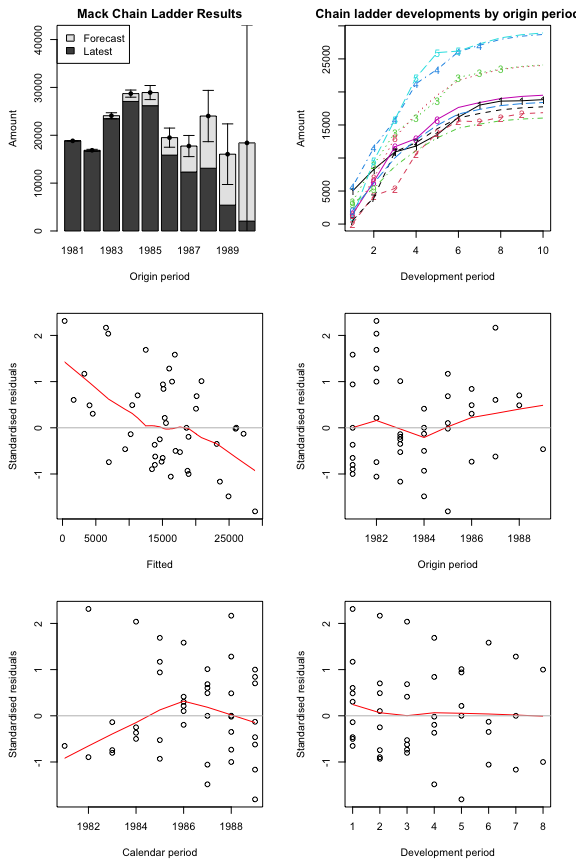Some residual show clear trends, indicating that the Mack assumptions are not well met We can plot the development, including the forecast and estimated standard errors by origin period by setting the argument lattice=TRUE. plot(mack, lattice=TRUE)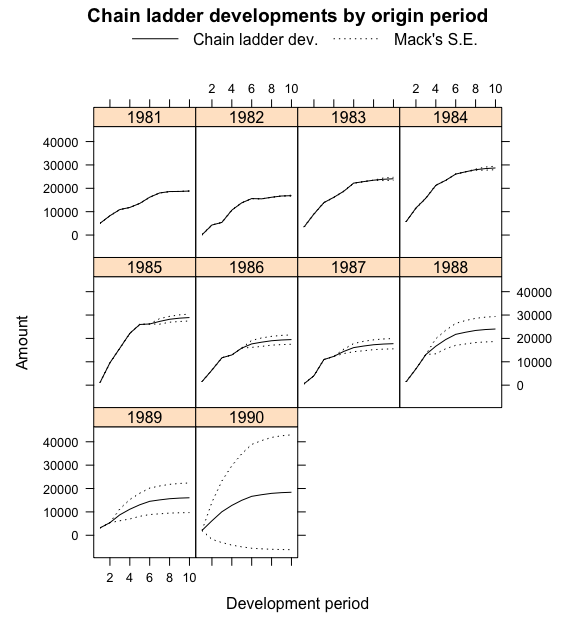#### Using a subset of the triangle The weights argument allows for the selection of a subset of the triangle for the projections. For example, in order to use only the last 5 calendar years of the triangle, set the weights as follows: calPeriods <- (row(RAA) + col(RAA) - 1) (weights <- ifelse(calPeriods <= 5, 0, ifelse(calPeriods > 10, NA, 1)))  [,1] [,2] [,3] [,4] [,5] [,6] [,7] [,8] [,9] [,10] [1,] 0 0 0 0 0 1 1 1 1 1 [2,] 0 0 0 0 1 1 1 1 1 NA [3,] 0 0 0 1 1 1 1 1 NA NA [4,] 0 0 1 1 1 1 1 NA NA NA [5,] 0 1 1 1 1 1 NA NA NA NA [6,] 1 1 1 1 1 NA NA NA NA NA [7,] 1 1 1 1 NA NA NA NA NA NA [8,] 1 1 1 NA NA NA NA NA NA NA [9,] 1 1 NA NA NA NA NA NA NA NA [10,] 1 NA NA NA NA NA NA NA NA NA MackChainLadder(RAA, weights=weights, est.sigma = "Mack") MackChainLadder(Triangle = RAA, weights = weights, est.sigma = "Mack") Latest Dev.To.Date Ultimate IBNR Mack.S.E CV(IBNR) 1981 18,834 1.0000 18,834 0 0 NaN 1982 16,704 0.9909 16,858 154 206 1.339 1983 23,466 0.9744 24,083 617 623 1.010 1984 27,067 0.9430 28,703 1,636 747 0.457 1985 26,180 0.9050 28,927 2,747 1,469 0.535 1986 15,852 0.8229 19,264 3,412 2,039 0.598 1987 12,314 0.7106 17,329 5,015 2,144 0.428 1988 13,112 0.5613 23,361 10,249 4,043 0.395 1989 5,395 0.2935 18,384 12,989 5,931 0.457 1990 2,063 0.0843 24,463 22,400 16,779 0.749 Totals Latest: 160,987.00 Dev: 0.73 Ultimate: 220,207.63 IBNR: 59,220.63 Mack.S.E 19,859.00 CV(IBNR): 0.34 ### Munich chain-ladder Munich chain-ladder is a reserving method that reduces the gap between IBNR projections based on paid losses and IBNR projections based on incurred losses. The Munich chain-ladder method uses correlations between paid and incurred losses of the historical data into the projection for the future (Quarg and Mack 2004). MCLpaid  dev origin 1 2 3 4 5 6 7 1 576 1804 1970 2024 2074 2102 2131 2 866 1948 2162 2232 2284 2348 NA 3 1412 3758 4252 4416 4494 NA NA 4 2286 5292 5724 5850 NA NA NA 5 1868 3778 4648 NA NA NA NA 6 1442 4010 NA NA NA NA NA 7 2044 NA NA NA NA NA NA MCLincurred  dev origin 1 2 3 4 5 6 7 1 978 2104 2134 2144 2174 2182 2174 2 1844 2552 2466 2480 2508 2454 NA 3 2904 4354 4698 4600 4644 NA NA 4 3502 5958 6070 6142 NA NA NA 5 2812 4882 4852 NA NA NA NA 6 2642 4406 NA NA NA NA NA 7 5022 NA NA NA NA NA NA par(mfrow=c(1,2)) plot(MCLpaid) plot(MCLincurred)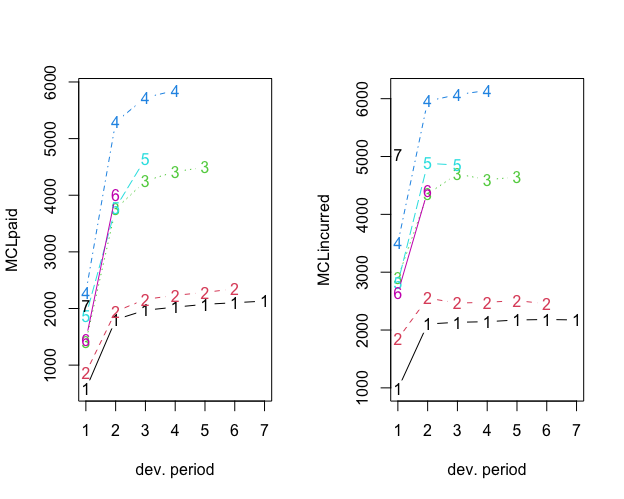par(mfrow=c(1,1)) # Following the example in Quarg's (2004) paper: MCL <- MunichChainLadder(MCLpaid, MCLincurred, est.sigmaP=0.1, est.sigmaI=0.1) MCL MunichChainLadder(Paid = MCLpaid, Incurred = MCLincurred, est.sigmaP = 0.1, est.sigmaI = 0.1) Latest Paid Latest Incurred Latest P/I Ratio Ult. Paid Ult. Incurred 1 2,131 2,174 0.980 2,131 2,174 2 2,348 2,454 0.957 2,383 2,444 3 4,494 4,644 0.968 4,597 4,629 4 5,850 6,142 0.952 6,119 6,176 5 4,648 4,852 0.958 4,937 4,950 6 4,010 4,406 0.910 4,656 4,665 7 2,044 5,022 0.407 7,549 7,650 Ult. P/I Ratio 1 0.980 2 0.975 3 0.993 4 0.991 5 0.997 6 0.998 7 0.987 Totals Paid Incurred P/I Ratio Latest: 25,525 29,694 0.86 Ultimate: 32,371 32,688 0.99 You can use summary(MCL)$ByOrigin and summary(MCL)$Totals to extract the information from the output above. plot(MCL)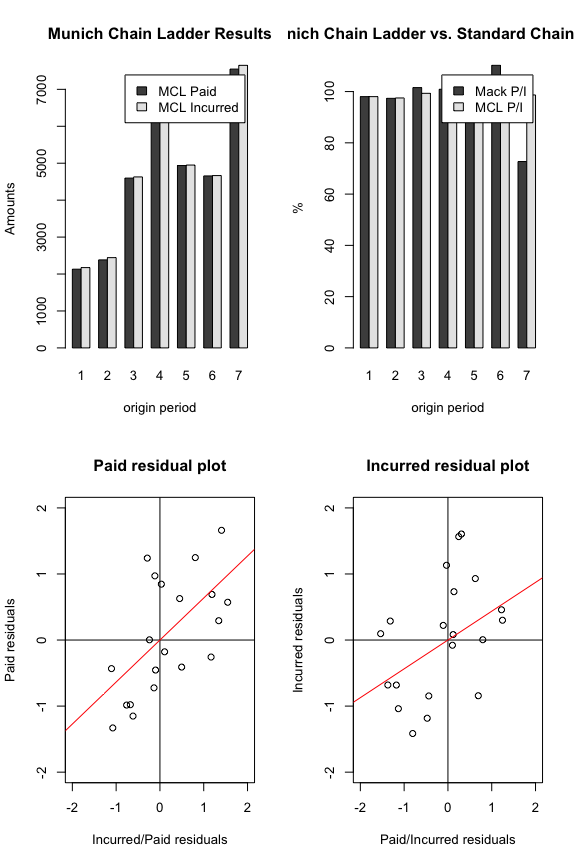### Bootstrap chain-ladder The BootChainLadder function uses a two-stage bootstrapping/simulation approach following the paper by England and Verrall (England and Verrall 2002). In the first stage an ordinary chain-ladder methods is applied to the cumulative claims triangle. From this we calculate the scaled Pearson residuals which we bootstrap R times to forecast future incremental claims payments via the standard chain-ladder method. In the second stage we simulate the process error with the bootstrap value as the mean and using the process distribution assumed. The set of reserves obtained in this way forms the predictive distribution, from which summary statistics such as mean, prediction error or quantiles can be derived. ## See also the example in section 8 of England & Verrall (2002) ## on page 55. B <- BootChainLadder(RAA, R=999, process.distr="gamma") B BootChainLadder(Triangle = RAA, R = 999, process.distr = "gamma") Latest Mean Ultimate Mean IBNR IBNR.S.E IBNR 75% IBNR 95% 1981 18,834 18,834 0 0 0 0 1982 16,704 16,860 156 657 188 1,401 1983 23,466 24,055 589 1,289 1,096 2,972 1984 27,067 28,653 1,586 1,904 2,524 5,006 1985 26,180 28,921 2,741 2,262 3,931 7,087 1986 15,852 19,594 3,742 2,560 5,157 8,548 1987 12,314 17,690 5,376 3,054 7,039 11,342 1988 13,112 24,096 10,984 5,003 13,865 20,353 1989 5,395 16,458 11,063 6,032 14,455 22,939 1990 2,063 19,325 17,262 14,236 24,829 42,957 Totals Latest: 160,987 Mean Ultimate: 214,486 Mean IBNR: 53,499 IBNR.S.E 19,669 Total IBNR 75%: 64,966 Total IBNR 95%: 87,510 You can use summary(B)$ByOrigin and summary(B)$Totals to extract the information from the output above. plot(B)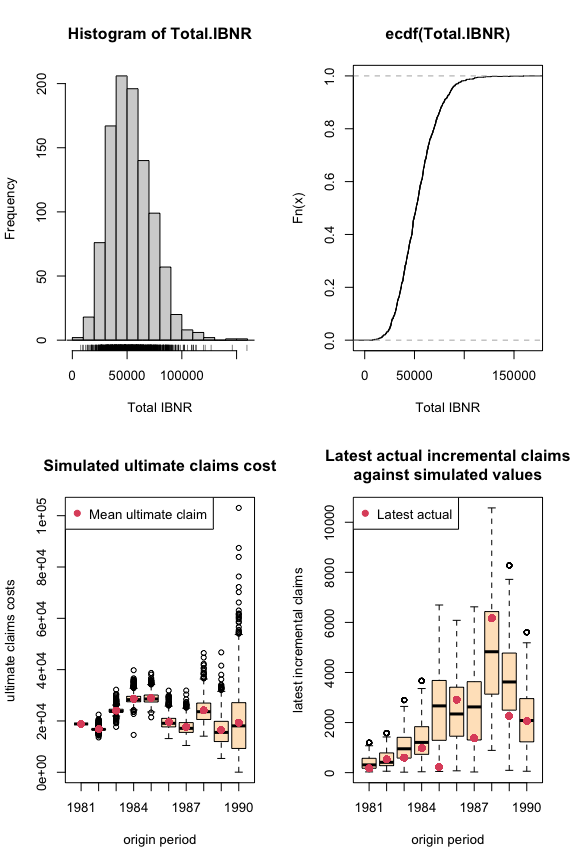Quantiles of the bootstrap IBNR can be calculated via the quantile function: quantile(B, c(0.75,0.95,0.99, 0.995)) $ByOrigin
IBNR 75% IBNR 95% IBNR 99% IBNR 99.5%
1981      0.0        0        0          0
1982    187.7     1401     2836       3360
1983   1095.8     2972     4826       5986
1984   2524.3     5006     7877       8476
1985   3930.9     7087     9384       9677
1986   5157.4     8548    12149      13497
1987   7038.6    11342    14018      14400
1988  13865.5    20353    25128      27401
1989  14455.0    22939    27620      28518
1990  24828.7    42957    59194      68156

$Totals Totals IBNR 75%: 64966 IBNR 95%: 87510 IBNR 99%: 108973 IBNR 99.5%: 113546 The distribution of the IBNR appears to follow a log-normal distribution, so let’s fit it: ## fit a distribution to the IBNR library(MASS) plot(ecdf(B$IBNR.Totals))
## fit a log-normal distribution
fit <- fitdistr(B$IBNR.Totals[B$IBNR.Totals>0], "lognormal")
fit
    meanlog      sdlog
10.816072    0.391640
( 0.012391) ( 0.008762)
curve(plnorm(x,fit$estimate["meanlog"], fit$estimate["sdlog"]),
col="red", add=TRUE)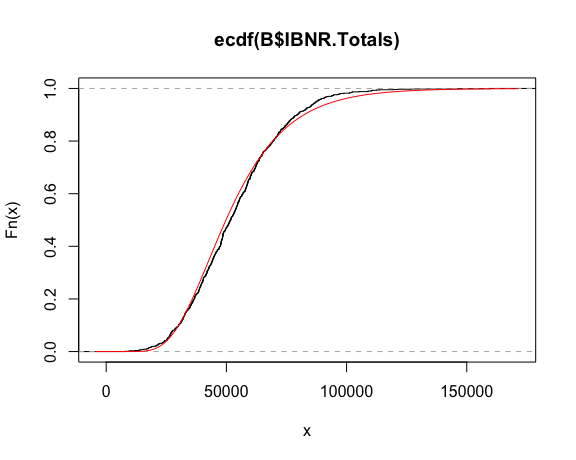The Mack chain-ladder technique can be generalized to the multivariate setting where multiple reserving triangles are modelled and developed simultaneously. The advantage of the multivariate modelling is that correlations among different triangles can be modelled, which will lead to more accurate uncertainty assessments. Reserving methods that explicitly model the between-triangle contemporaneous correlations can be found in (Pröhl and Schmidt 2005), (Michael Merz and Wüthrich 2008b). Another benefit of multivariate loss reserving is that structural relationships between triangles can also be reflected, where the development of one triangle depends on past losses from other triangles. For example, there is generally need for the joint development of the paid and incurred losses (Quarg and Mack 2004). Most of the chain-ladder-based multivariate reserving models can be summarised as sequential seemingly unrelated regressions (Zhang 2010). We note another strand of multivariate loss reserving builds a hierarchical structure into the model to allow estimation of one triangle to “borrow strength” from other triangles, reflecting the core insight of actuarial credibility (Zhang, Dukic, and Guszcza 2012).

Denote $$Y_{i,k}=(Y^{(1)}_{i,k}, \cdots ,Y^{(N)}_{i,k})$$ as an $$N \times 1$$ vector of cumulative losses at accident year $$i$$ and development year $$k$$ where $$(n)$$ refers to the n-th triangle. (Zhang 2010) specifies the model in development period $$k$$ as:

$\begin{equation} Y_{i,k+1} = A_k + B_k \cdot Y_{i,k} + \epsilon_{i,k}, \end{equation}$

where $$A_k$$ is a column of intercepts and $$B_k$$ is the development matrix for development period $$k$$. Assumptions for this model are:

\begin{aligned} &E(\epsilon_{i,k}|Y_{i,1}, \cdots,Y_{i,I+1-k}) =0, \\ &cov(\epsilon_{i,k}|Y_{i,1}, \cdots, Y_{i,I+1-k})=D(Y_{i,k}^{-\delta/2}) \, \Sigma_k \, D(Y_{i,k}^{-\delta/2}), \\ &\text{losses of different accident years are independent}, \\ &\epsilon_{i,k} \text{ are symmetrically distributed}. \end{aligned}

In the above, $$D$$ is the diagonal operator, and $$\delta$$ is a known positive value that controls how the variance depends on the mean (as weights). This model is referred to as the general multivariate chain ladder [GMCL] in (Zhang 2010). A important special case where $$A_k=0$$ and $$B_k$$’s are diagonal is a naive generalization of the chain-ladder, often referred to as the multivariate chain-ladder [MCL] (Pröhl and Schmidt 2005).

In the following, we first introduce the class triangles, for which we have defined several utility functions. Indeed, any input triangles to the MultiChainLadder function will be converted to triangles internally. We then present loss reserving methods based on the MCL and GMCL models in turn.

Consider the two liability loss triangles from (Michael Merz and Wüthrich 2008b). It comes as a list of two matrices:

str(liab)
List of 2
$GeneralLiab: num [1:14, 1:14] 59966 49685 51914 84937 98921 ...$ AutoLiab   : num [1:14, 1:14] 114423 152296 144325 145904 170333 ...

We can convert a list to a triangles object using

liab2 <- as(liab, "triangles")
class(liab2)
 "triangles"
attr(,"package")
 "ChainLadder"

We can find out what methods are available for this class:

showMethods(classes = "triangles")

For example, if we want to extract the last three columns of each triangle, we can use the [ operator as follows:

# use drop = TRUE to remove rows that are all NA's
liab2[, 12:14, drop = TRUE]
An object of class "triangles"
[]
[,1]   [,2]   [,3]
[1,] 540873 547696 549589
[2,] 563571 562795     NA
[3,] 602710     NA     NA

[]
[,1]   [,2]   [,3]
[1,] 391328 391537 391428
[2,] 485138 483974     NA
[3,] 540742     NA     NA

The following combines two columns of the triangles to form a new matrix:

cbind2(liab2[1:3, 12])
       [,1]   [,2]
[1,] 540873 391328
[2,] 563571 485138
[3,] 602710 540742

The form of regression models used in estimating the development parameters is controlled by the fit.method argument. If we specify fit.method = "OLS", the ordinary least squares will be used and the estimation of development factors for each triangle is independent of the others. In this case, the residual covariance matrix $$\Sigma_k$$ is diagonal. As a result, the multivariate model is equivalent to running multiple Mack chain-ladders separately.

fit1 <- MultiChainLadder(liab, fit.method = "OLS")
lapply(summary(fit1)$report.summary, "[", 15, ) $Summary Statistics for Triangle 1
Latest Dev.To.Date Ultimate    IBNR    S.E     CV
Total 11343397      0.6482 17498658 6155261 427289 0.0694

$Summary Statistics for Triangle 2 Latest Dev.To.Date Ultimate IBNR S.E CV Total 8759806 0.8093 10823418 2063612 162872 0.0789$Summary Statistics for Triangle 1+2
Latest Dev.To.Date Ultimate    IBNR    S.E     CV
Total 20103203      0.7098 28322077 8218874 457278 0.0556

In the above, we only show the total reserve estimate for each triangle to reduce the output. The full summary including the estimate for each year can be retrieved using the usual summary function. By default, the summary function produces reserve statistics for all individual triangles, as well as for the portfolio that is assumed to be the sum of the two triangles. This behaviour can be changed by supplying the portfolio argument. See the documentation for details.

We can verify if this is indeed the same as the univariate Mack chain ladder. For example, we can apply the MackChainLadder function to each triangle:

fit <- lapply(liab, MackChainLadder, est.sigma = "Mack")
# the same as the first triangle above
lapply(fit, function(x) t(summary(x)$Totals)) $GeneralLiab
Latest:   Dev: Ultimate:   IBNR: Mack S.E.: CV(IBNR):
Totals 11343397 0.6482  17498658 6155261     427289   0.06942

$AutoLiab Latest: Dev: Ultimate: IBNR: Mack S.E.: CV(IBNR): Totals 8759806 0.8093 10823418 2063612 162872 0.07893 The argument mse.method controls how the mean square errors are computed. By default, it implements the Mack method. An alternative method is the conditional re-sampling approach in (Buchwalder et al. 2006), which assumes the estimated parameters are independent. This is used when mse.method = "Independence". For example, the following reproduces the result in (Buchwalder et al. 2006). Note that the first argument must be a list, even though only one triangle is used. (B1 <- MultiChainLadder(list(GenIns), fit.method = "OLS", mse.method = "Independence")) $Summary Statistics for Input Triangle
Latest Dev.To.Date   Ultimate       IBNR       S.E    CV
1      3,901,463      1.0000  3,901,463          0         0 0.000
2      5,339,085      0.9826  5,433,719     94,634    75,535 0.798
3      4,909,315      0.9127  5,378,826    469,511   121,700 0.259
4      4,588,268      0.8661  5,297,906    709,638   133,551 0.188
5      3,873,311      0.7973  4,858,200    984,889   261,412 0.265
6      3,691,712      0.7223  5,111,171  1,419,459   411,028 0.290
7      3,483,130      0.6153  5,660,771  2,177,641   558,356 0.256
8      2,864,498      0.4222  6,784,799  3,920,301   875,430 0.223
9      1,363,294      0.2416  5,642,266  4,278,972   971,385 0.227
10       344,014      0.0692  4,969,825  4,625,811 1,363,385 0.295
Total 34,358,090      0.6478 53,038,946 18,680,856 2,447,618 0.131

### Multivariate chain-ladder using seemingly unrelated regressions

To allow correlations to be incorporated, we employ the seemingly unrelated regressions (see the package systemfit, (Henningsen and Hamann 2007)) that simultaneously model the two triangles in each development period. This is invoked when we specify fit.method = "SUR":

fit2 <- MultiChainLadder(liab, fit.method = "SUR")
lapply(summary(fit2)$report.summary, "[", 15, ) $Summary Statistics for Triangle 1
Latest Dev.To.Date Ultimate    IBNR    S.E     CV
Total 11343397      0.6484 17494907 6151510 419293 0.0682

$Summary Statistics for Triangle 2 Latest Dev.To.Date Ultimate IBNR S.E CV Total 8759806 0.8095 10821341 2061535 162464 0.0788$Summary Statistics for Triangle 1+2
Latest Dev.To.Date Ultimate    IBNR    S.E    CV
Total 20103203        0.71 28316248 8213045 500607 0.061

We see that the portfolio prediction error is inflated to $$500,607$$ from $$457,278$$ in the separate development model (“OLS”). This is because of the positive correlation between the two triangles. The estimated correlation for each development period can be retrieved through the residCor function:

round(unlist(residCor(fit2)), 3)
   0.247  0.495  0.682  0.446  0.487  0.451 -0.172  0.805  0.337  0.688
 -0.004  1.000  0.021

Similarly, most methods that work for linear models such as coef, fitted, resid and so on will also work. Since we have a sequence of models, the retrieved results from these methods are stored in a list. For example, we can retrieve the estimated development factors for each period as

do.call("rbind", coef(fit2))
      eq1_x[] eq2_x[]
[1,]      3.227     2.2224
[2,]      1.719     1.2688
[3,]      1.352     1.1200
[4,]      1.179     1.0665
[5,]      1.106     1.0356
[6,]      1.055     1.0168
[7,]      1.026     1.0097
[8,]      1.015     1.0002
[9,]      1.012     1.0038
[10,]      1.006     0.9994
[11,]      1.005     1.0039
[12,]      1.005     0.9989
[13,]      1.003     0.9997

The smaller-than-one development factors after the 10-th period for the second triangle indeed result in negative IBNR estimates for the first several accident years in that triangle.

The package also offers the plot method that produces various summary and diagnostic figures: